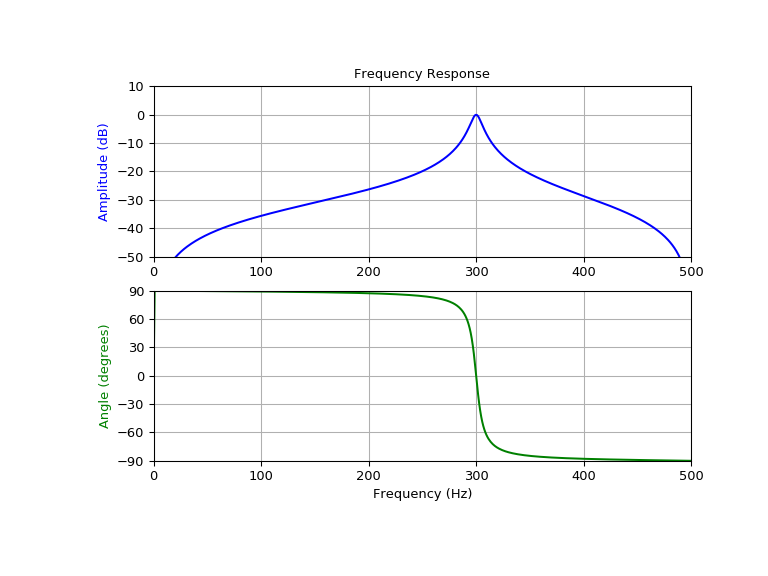# scipy.signal.iirpeak¶

scipy.signal.iirpeak(w0, Q, fs=2.0)[source]

Design second-order IIR peak (resonant) digital filter.

A peak filter is a band-pass filter with a narrow bandwidth (high quality factor). It rejects components outside a narrow frequency band.

Parameters
w0float

Frequency to be retained in a signal. If fs is specified, this is in the same units as fs. By default, it is a normalized scalar that must satisfy 0 < w0 < 1, with w0 = 1 corresponding to half of the sampling frequency.

Qfloat

Quality factor. Dimensionless parameter that characterizes peak filter -3 dB bandwidth bw relative to its center frequency, Q = w0/bw.

fsfloat, optional

The sampling frequency of the digital system.

New in version 1.2.0.

Returns
b, andarray, ndarray

Numerator (b) and denominator (a) polynomials of the IIR filter.

Notes

New in version 0.19.0.

References

1

Sophocles J. Orfanidis, “Introduction To Signal Processing”, Prentice-Hall, 1996

Examples

Design and plot filter to remove the frequencies other than the 300 Hz component from a signal sampled at 1000 Hz, using a quality factor Q = 30

>>> from scipy import signal
>>> import matplotlib.pyplot as plt

>>> fs = 1000.0  # Sample frequency (Hz)
>>> f0 = 300.0  # Frequency to be retained (Hz)
>>> Q = 30.0  # Quality factor
>>> # Design peak filter
>>> b, a = signal.iirpeak(f0, Q, fs)

>>> # Frequency response
>>> freq, h = signal.freqz(b, a, fs=fs)
>>> # Plot
>>> fig, ax = plt.subplots(2, 1, figsize=(8, 6))
>>> ax.plot(freq, 20*np.log10(np.maximum(abs(h), 1e-5)), color='blue')
>>> ax.set_title("Frequency Response")
>>> ax.set_ylabel("Amplitude (dB)", color='blue')
>>> ax.set_xlim([0, 500])
>>> ax.set_ylim([-50, 10])
>>> ax.grid()
>>> ax.plot(freq, np.unwrap(np.angle(h))*180/np.pi, color='green')
>>> ax.set_ylabel("Angle (degrees)", color='green')
>>> ax.set_xlabel("Frequency (Hz)")
>>> ax.set_xlim([0, 500])
>>> ax.set_yticks([-90, -60, -30, 0, 30, 60, 90])
>>> ax.set_ylim([-90, 90])
>>> ax.grid()
>>> plt.show()#### Previous topic

scipy.signal.iirnotch

scipy.signal.lti